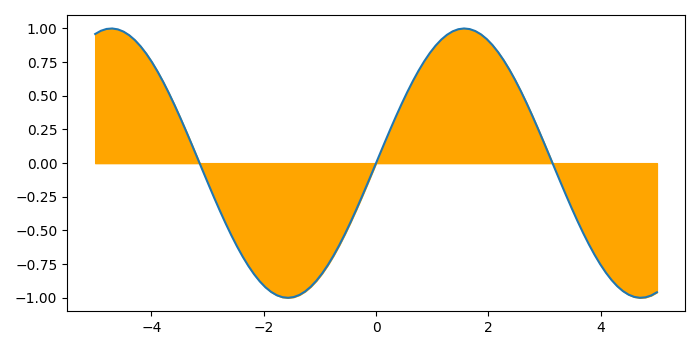# Filling the region between a curve and X-axis in Python using Matplotlib

MatplotlibPythonData Visualization

To fill the region between a curve and X-axis in Python using Matplotlib, we can take the following steps

## Steps

• Set the figure size and adjust the padding between and around the subplots.

• Create x and y data points using numpy.

• Plot the x and y data points using plot() method.

• Fill the area between the curve and the X-axis using fill_between() method.

• To display the figure, use Show() method.

## Example

import matplotlib.pyplot as plt
import numpy as np

# Set the figure size
plt.rcParams["figure.figsize"] = [7.00, 3.50]
plt.rcParams["figure.autolayout"] = True

# Create x and y data points
x = np.linspace(-5, 5, 100)
y = np.sin(x)

# Plot the x and y data points
plt.plot(x, y)

# Fill the region with color
plt.fill_between(x, 0, y, color='orange')

# Display the plot
plt.show()


## Output

It will produce the following output −